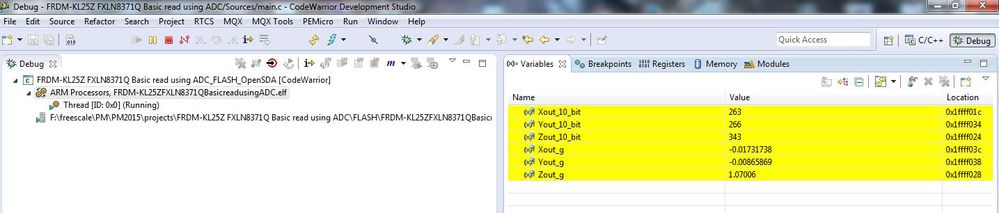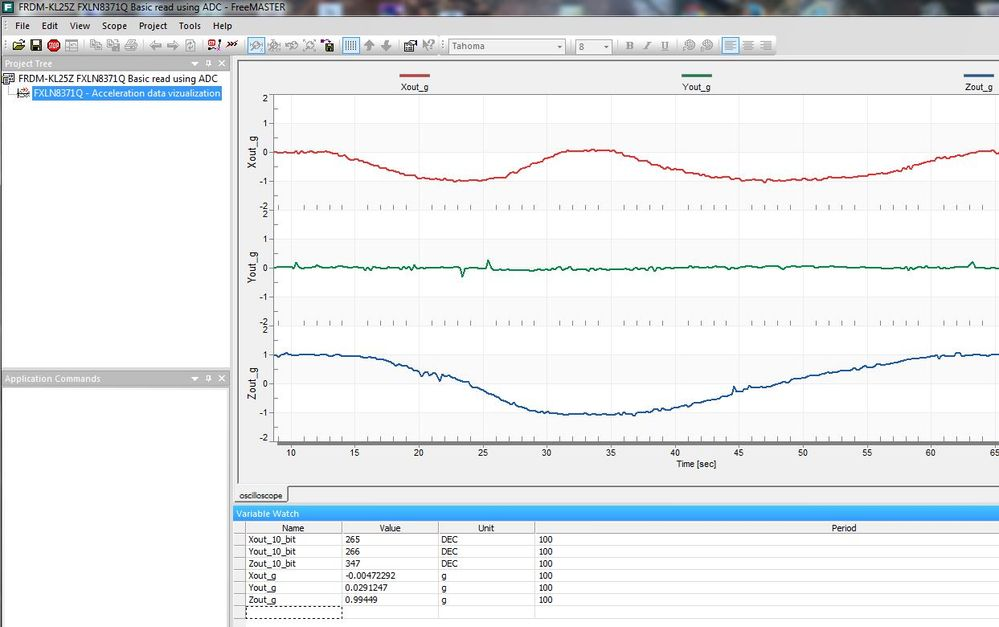# FXLN8371Q - Bare metal example project

cancel
Showing results for
Did you mean:

# FXLN8371Q - Bare metal example project

No ratings

## FXLN8371Q - Bare metal example project

Hi Everyone,

In this document I would like to present a simple bare-metal example code/demo for the FXLN8371Q Xtrinsic three axis, low-power, low-g, analog output accelerometer. I have created it while working with the Freescale FRDM-KL25Z development platform and FXLN8371Q breakout board. The FreeMASTER tool is used to visualize the acceleration data that are read from the FXLN8371Q through ADC.

This example illustrates:

1. Initialization of the MKL25Z128 MCU (mainly ADC, PORT and PIT modules).

2. Simple offset calibration.

3. Accelerometer outputs reading using ADC and conversion of the 10-bit ADC values to real acceleration values in g’s.

4. Visualization of the output values in the FreeMASTER tool.

1. The FXLN8371Q breakout board (schematic is attached below) needs to have the following pins connected to the FRDM-KL25Z board:

J4-1 (VDD) => J9-4 (P3V3)

J4-4 (ZOUT) => J10-6 (PTB2/ ADC0_SE12)

J4-6 (GND) => J9-14 (GND)

J3-3 (ST) => J9-14 (GND)

J3-4 (EN) => J9-4 (P3V3)

The PIT (Periodic Interrupt Timer) is used to read the output data periodically at a fixed rate of ~200Hz. The MCU is, therefore, configured as follows.

void MCU_Init(void)

{

SIM_SCGC5 |= SIM_SCGC5_PORTB_MASK;       // Turn on clock to Port B module

PORTB_PCR0 |= PORT_PCR_MUX(0x00);        // PTB0 pin is ADC0 SE8 input

PORTB_PCR1 |= PORT_PCR_MUX(0x00);        // PTB1 pin is ADC0 SE9 input

PORTB_PCR2 |= PORT_PCR_MUX(0x00);        // PTB2 pin is ADC0 SE12 input

//PIT module initialization

SIM_SCGC6 |= SIM_SCGC6_PIT_MASK;         // Turn on clock to PIT module

PIT_LDVAL0 = 52400;                      // Timeout period = ~5ms (200Hz)

PIT_MCR = PIT_MCR_FRZ_MASK;              // Enable clock for PIT, freeze PIT in debug mode

//Enable PIT interrupt on NVIC

NVIC_ICPR |= 1 << ((INT_PIT - 16) % 32);

NVIC_ISER |= 1 << ((INT_PIT - 16) % 32);

}

2. The simplest offset calibration method consists of placing the board on a flat surface so that X is at 0g, Y is at 0g and Z is at +1g. 16 samples are recorded and then averaged. The known sensitivity needs to be subtracted from the +1g reading to calculate an assumed 0g offset value for Z.

void Calibrate(void)

{

unsigned int Count = 0;

do                   // Accumulate 16 samples for X, Y, Z

{

Count++;

} while (Count < 16);

X_offset = (float) Xout_10_bit / 16;                             // Compute X-axis offset by averaging all 16 X-axis samples

Y_offset = (float) Yout_10_bit / 16;                             // Compute Y-axis offset by averaging all 16 Y-axis samples

Z_offset = ((float) Zout_10_bit / 16) - SENSITIVITY_2G;          // Compute Z-axis offset by averaging all 16 Z-axis samples and subtracting the known sensitivity

}

229 mV/g = 229 mV/g x (1023 ADC units) / 2.95V = 79.4 ADC units/g = SENSITIVITY_2g

Using this calculated sensitivity and measured offset, I convert the 10-bit ADC values to real acceleration values in g’s in the PIT ISR as follows.

void PIT_IRQHandler()

{

Xout_g = ((float) Xout_10_bit - X_offset) / SENSITIVITY_2G;         // Compute X-axis output value in g's

Yout_g = ((float) Yout_10_bit - Y_offset) / SENSITIVITY_2G;         // Compute Y-axis output value in g's

Zout_g = ((float) Zout_10_bit - Z_offset) / SENSITIVITY_2G;         // Compute Z-axis output value in g's

PIT_TFLG0 |= PIT_TFLG_TIF_MASK;          // Clear PIT interrupt flag

}

4. The calculated values can be watched in the "Variables" window on the top right of the Debug perspective or in the FreeMASTER application. To open and run the FreeMASTER project, install the FreeMASTER application and FreeMASTER Communication Driver.I guess this is enough to let you start experimenting with the FXLN83xxQ family of analog accelerometers. Attached you can find the complete source code written in the CW for MCU's v10.6 including the FreeMASTER project.

If there are any questions regarding this simple application, do not hesitate to ask below. Your feedback or suggestions are also welcome.

Regards,

Tomas